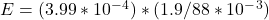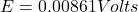## A very long solenoid with a circular cross section and radius r1= 1.30 cm with ns= 260 turns/cm lies inside a short coil of radius r2= 4.60

Question

A very long solenoid with a circular cross section and radius r1= 1.30 cm with ns= 260 turns/cm lies inside a short coil of radius r2= 4.60 cm and Nc= 23 turns. If the current in the solenoid is ramped at a constant rate from zero to Is= 1.90 A over a time interval of 88.0 ms, what is the magnitude of the emf in the outer coil while the current in the solenoid is changing?

in progress 0
2 months 2021-08-04T22:27:19+00:00 1 Answers 3 views 0

0.00851 volts

Explanation:

radius r1= 1.30 cm with ns= 260 turns/cm

radius r2= 4.60 cm and Nc= 23 turns

constant rate from zero to Is= 1.90 A

time interval of 88.0 ms

Area of the solenoid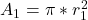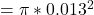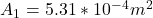Mututal Inductance

M=uo*n*N*A1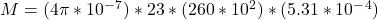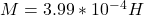a)

EMF induced in the outer coil

E=M(dIs/dt)